# GSEB Solutions Class 9 Maths Chapter 10 Circles Ex 10.5

Gujarat Board GSEB Solutions Class 9 Maths Chapter 10 Circles Ex 10.5 Textbook Questions and Answers.

## Gujarat Board Textbook Solutions Class 9 Maths Chapter 10 Circles Ex 10.5

Question 1.
Infigure,A,B and Carethreepointsona circle with centre O such that ∠BOC = 300 and ∠AOB = 60°. If D is a point on the circle other than the arc ABC, find ∠ADC.Solution:
∠ADC = $$\frac {1}{2}$$∠AOC
[The angle subtended by an arc at the centre is double the angle subtended by it any point on the remaining part of the circle.]
= $$\frac {1}{2}$$(∠AOB + ∠BOC)
= $$\frac {1}{2}$$ (60° + 300)
= $$\frac {1}{2}$$ (90°) = 45°

Question 2.
A chord of a circle is equal to the radius of the circle. Find the angle subtended by the chord at a point on the minor arc and also at a point on the major arc.
Solution:
∴ OA = OB = AB [Given]
∴ OAB is equilateral.
∴ ∠AOB = 60°
∠ACB = $$\frac {1}{2}$$∠A0B
[The angle subtended by an arc at the centre is double the angle subtended by it at any point on the remaining part of the circle.]
∴ ∠ACB = $$\frac {1}{2}$$ x 60° = 30°∴ ∠ADB + ∠ACB = 180°
[The sum of either pair of opposite angles of a cyclic quadrilateral is 180°]Question 3.
In figure, ∠PQR = 100°, where P, Q and R are points on a circle with centre O. Find ∠OPR.Solution:
Take a point S in the major arc. Join PS and RS.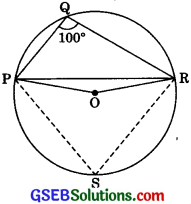∴ PQRS is a cyclic quadrilateral.
∠PQR + ∠PSR = 180°
The sum of either pair of opposite angles of a cyclic quadrilateral is 180°
100° + ∠PSR = 180°
∠PSR = 180° – 100°
⇒  ∠PSR = 80° …….(1)
Now, ∠POR = 2∠PSR
(The angle subtended by an arc at the centre is double the angle subtended by it at any point on the remaining part of the circle]
∴ ∠POR= 2 x 800 = 160° …….(2) Using (1)In ΔOPR,
∴ ∠OPR = ∠ORP …………(3)
Angles opposite to equal sides of a triangle are equal
Also,
∠OPR + ∠ORP + ∠POR = 180°
Sum of all the angles of a triangle is 1800
⇒ ∠OPR + ∠OPR + 160° = 180°
Using (2) and (1)
⇒ 2∠OPR + 160° = 180°
⇒ 2∠OPR = 180° – 160° = 20°
∠OPR = 10°

Question 4.
In figure, ∠ABC = 69°, ∠ACB = 31°, find ∠BDC.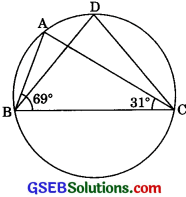Solution:
In ΔABC,
∠BAC + ∠ABC + ∠ACB = 180°
[Sum of all the angles of a triangle is 180°]
∠BAC + 69° + 31° = 180°
∠BAC + 100° = 1800
∠BAC = 180° = 100° = 80° ………..(1)
Now, ∠BDC = ∠BAC
[Angles in the same segment of a circle are equal]
∠BDC = 80° [Using (1)]Question 5.
In figure, A, B, C and D are four points on a circle. AC and BD intersect at a point E such that ∠BEC = 130° and ∠ECD = 20°. Find ∠BAC.Solution:
In ΔCDE ∠CDE + ∠DCE = ∠BEC
[Exterior angle property of a triangle]
⇒ ∠CDE + 20° = 130°

⇒ ∠CDE = 130° – 20° = 110° ………..(2)
Now, ∠BAC = ∠CDE
[Angles in the same segment of a circle are equal]
∴ ∠BAC = ∠110° [Usmg(1)]

Question 6.
ABCD is a cyclic quadrilateral whose diagonals intersect at a point E. If ∠DBC = 70°, ∠BAC is 30°, find ∠BCD. Further, If AB = BC, find ∠ECD.
Solution:
∠CDB = ∠BAC
[Angles in the same segment of a circle are equal]
∠CDB = 30° ………..(1)
∠DBC = 70° ………..(2) (Given)
In ΔBCD,∠BCD + ∠DBC + ∠CDB = 180°
(Sum of all the angles of a triangle is 180°]
⇒ ∠BCD + 70° + 300 = 180° [Using (1) and (2)1
⇒ ∠BCD + 100° = 180°
∠BCD = 180° – 100°
⇒ ∠BCD = 80° ………(3)
In ΔABC, AB = BC
∠BCA = ∠BAC
[Angles opposite to equal sides of a triangle are equal]
⇒ ∠BCA = 30° ………(4)
∠BAC = 30° (given]
Now, ∠BCD = 80° (From (3))
= ∠BCA + ∠ECD = 80°
⇒ 30°+∠ECD = 80°
⇒ ∠ECD = 80 – 30°
⇒ ∠ECD = 50°Question 7.
If diagonals of a cyclic quadrilateral are diameters of the circle through the vertices of the quadrilateral, prove that it is a rectangle.
Solution:
In ΔOAB and ΔOCD,
OA = OC [Radii of a circle]
OB = OD [Radii of a circle]
∠AOB = ∠COD [Vertically opposite angles]∴ ΔOAB = ΔOCD [SAS rule]
∴ AB = CD …….(1) [CPCTI
Similarly, we can show that
Adding (1) and (2), we get
ABCD is a || gm.
(A quadrilateral having opposite sides equal is a parallelogram)
Now, diagonal BD is also a diameter
∴ ∠BAD = $$\frac {1}{2}$$BOD
= $$\frac {1}{2}$$ x 180° = 90°
∴ ABCD is the rectangle. (A parallelogram with an angle 90° is a rectangel)Question 8.
If the non-parallel sides of a trapezium are equal, prove that it is cyclic. Prove that an isosceles trapezium is cyclic.
Solution:
Given: ABCD is a trapezium whose two non-parallel sides AD and BC are equal.
To Prove: Trapezium ABCD is cyclic.
Proof: AB || DE [Given]
∴ Quadrilateral ABED is a parallelogram.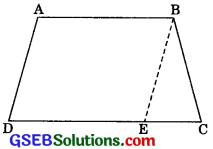∴ ∠BAD = ∠BED ……….(1) [Opp. ∠s of a || gm]
and AD = BE [Opp. sides of a gin]
But AD = BC ………(3) [Given]
From (2) and (3),
BE = BC
∴ ∠BEC = ∠BCE ………(4)
[Angles opposite to equal sides]
∠BEC + ∠BED = 1800 [ Linear Pair Axiom]
⇒ ∠BCE + ∠BAD = 1800 [From (4) and (1)]
⇒ Trapezium ABCD is cyclic.
[ If a pair of opposite angles of a quadrilateral is 180°, then the quadrilateral is cyclic.]Question 9.
Two circles intersect at two points B and C. Through B, two line segments ABD and PBQ are drawn to intersect the circles at A, D and P, Q respectively (see figure). Prove that
∠ACP = ∠QCD.Solution:
∠ACP = ∠ABP …………(1)
[Angles in the same segment of a circle are equal]
And ., ∠QCD = ∠QBD …………(2)
[Angles in the same segment of a circle are equal]
But ∠ABP = ∠QBD [Vertically opposite angles]
∠ACP = ∠QCD. [From (1) and (2)]Question 10.
If circles are drawn taking two sides of a triangle as diameter, prove that the point of intersection of these circles lies on the third side.
Solution:
Given: Circles are described with sides AB and AC of a triangle ABC as diameters. They intersect at a point D.
To Prove: D lies on the third side BC of ∠ABC.Proof: Circle described on AB as diameter intersects BC in D.
[Angle in a semicircle Similarly, the circle described on AC as diameter passes through D.]
Now, adding (1) and (2) we get
∴ Points B, D, C are collinear.
∴ D lies on BC.Question 11.
ABC and ADC are two right triangles with common hypotenuse AC. Prove that ∠CAD = ∠CBD.
Solution:
∴ AC is the common hypotenuse of two right triangles ABC and ADC.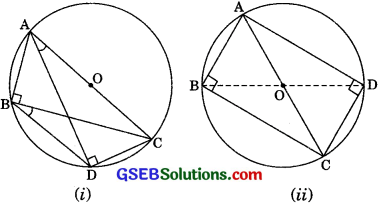∴ ∠ABC = 90° = ∠ADC
Here, there are two cases arising i.e., B and D are either on the same side of AC or on opposite sides.

Case I – Both the triangles are in the same semicircle.
∴ Points A, B, D and C are concyclic.

Case II – Triangles are on opposite sides, then
∴ A, B, C and D are concyclic.
Now, in both cases, DC is a chord
[∴ Angles in the same segment are equal]Question 12.
Prove that a cyclic parallelogram is a rectangle.
Solution:
Given: ABCD is a cyclic parallelogram.
To prove: ABCD is a rectangle.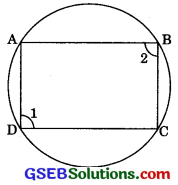Proof: ABCD is a cyclic quadrilateral.
∴ ∠1 + ∠2 = 180° ……….(1)
[∴ Opposite angles of a cyclic quadrilateral are supplementary]
∴ ABCD is a parailelograin.
∴ ∠1 = ∠2 ………(2) [Opp. angles of a || gm]
From (1) and (2),
∠1 = ∠2 = 90°
∴ || gm ABCD is a rectangle.